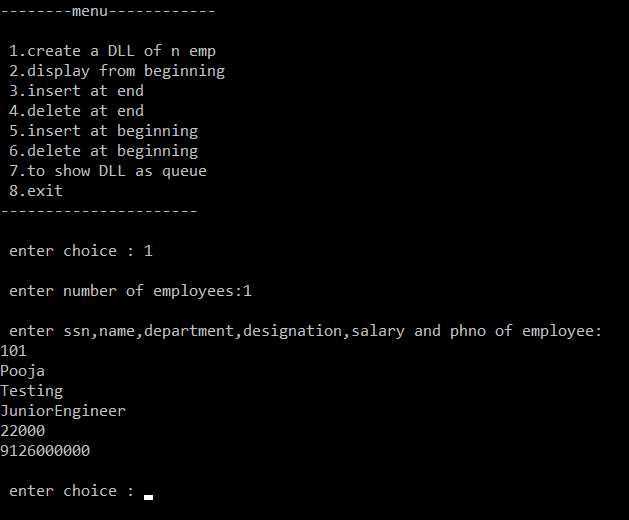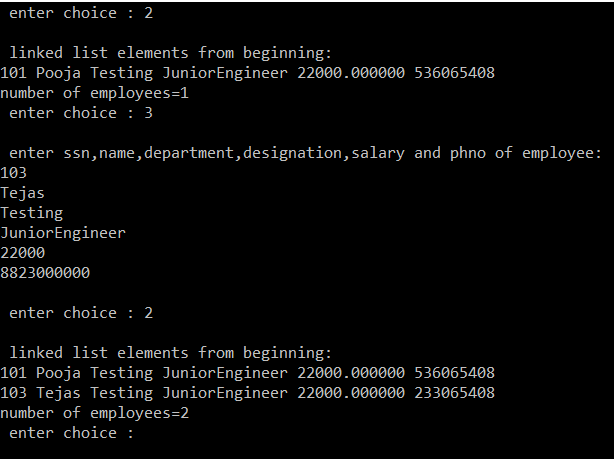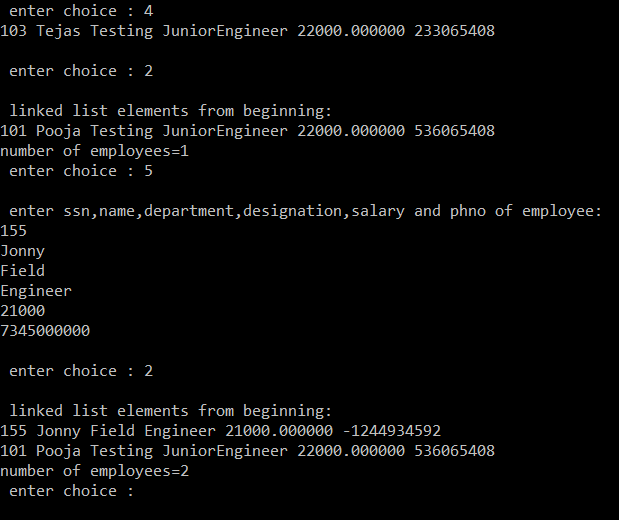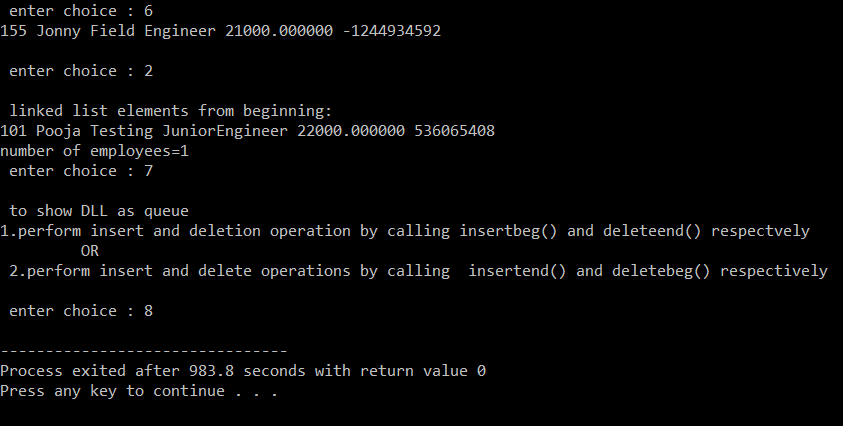Open in App
Not now

# Employee Management System using doubly linked list in C

• Difficulty Level : Medium
• Last Updated : 12 Oct, 2021

Design and implement a menu-driven program in C for the below operations on DLL of employee data with fields: SSN, name, department, designation, Salary, Phone Number:

• Create a DLL of N employee’s data by using end insertion.
• Display the status of DLL and count the number of nodes in it.
• Perform insertion and deletion at end of DLL.
• Perform insertion and deletion at front of DLL.
• Demonstrate how this DLL can be used as a double-ended queue.

Approach:

• For storing the data of the employee, create a user define datatype which will store the information regarding Employee. Below is the declaration of the data type:
```struct node {
struct node* prev;
int ssn;
long int phno;
float sal;
char name, dept, desg;
struct node* next;
}```
• Building the Employee’s table: For building the employee table the idea is to use the above struct datatype which will use to store the information regarding the employee and every new employee’s details will be added as a linked list node.
• Deleting in the record: Since, a doubly-linked list is used to store the data, therefore to delete the data at any index just link the next to the next of the deleted data and link the previous node of the next data of the deleted node to its previous data.
• Searching in the record: For searching in the record based on any parameter, the idea is to traverse the data and if at any index the value parameters match with the record stored, print all the information of that employee.

Below is the C-program to demonstrate employers details using a Doubly Linked List:

## C

 `// C-program to demonstrate employer``// details using a Doubly-linked list``#include ``#include ``#include ``#include `` ` `// Global declaration``int` `count = 0;`` ` `// Structure declaration``struct` `node {``    ``struct` `node* prev;``    ``int` `ssn;``    ``long` `int` `phno;``    ``float` `sal;``    ``char` `name, dept, desg;``    ``struct` `node* next;``} * h, *temp, *temp1, *temp2, *temp4;`` ` `// Function to create node``void` `create()``{``    ``int` `ssn;``    ``long` `int` `phno;``    ``float` `sal;``    ``char` `name, dept, desg;``    ``temp = (``struct` `node*)``malloc``(``sizeof``(``struct` `node));``    ``temp->prev = NULL;``    ``temp->next = NULL;``    ``printf``(``"\n enter ssn, name, depart"``           ``"ment, designation, salary "``           ``"and phno of employee:\n"``);``    ``scanf``(``"%d %s %s %s %f %ld"``,``          ``&ssn, name, dept, desg,``          ``&sal, &phno);``    ``temp->ssn = ssn;``    ``strcpy``(temp->name, name);``    ``strcpy``(temp->dept, dept);``    ``strcpy``(temp->desg, desg);``    ``temp->sal = sal;``    ``temp->phno = phno;``    ``count++;``}`` ` `// Function to insert at beginning``void` `insertbeg()``{``    ``// If DLL is empty``    ``if` `(h == NULL) {``        ``create();``        ``h = temp;``        ``temp1 = h;``    ``}`` ` `    ``// Else create a new node and``    ``// update the links``    ``else` `{``        ``create();``        ``temp->next = h;``        ``h->prev = temp;``        ``h = temp;``    ``}``}`` ` `// Function to insert at end``void` `insertend()``{``    ``// If DLL is empty``    ``if` `(h == NULL) {``        ``create();``        ``h = temp;``        ``temp1 = h;``    ``}`` ` `    ``// Else create a new node and``    ``// update the links``    ``else` `{``        ``create();``        ``temp1->next = temp;``        ``temp->prev = temp1;``        ``temp1 = temp;``    ``}``}`` ` `// Function to display from beginning``void` `displaybeg()``{``    ``temp2 = h;``    ``if` `(temp2 == NULL) {``        ``printf``(``"\n list is empty\n"``);``        ``return``;``    ``}``    ``printf``(``"\n linked list elements "``           ``"from beginning:\n"``);``    ``while` `(temp2 != NULL) {``        ``printf``(``"%d %s %s %s %f %ld\n"``,``               ``temp2->ssn, temp2->name,``               ``temp2->dept, temp2->desg,``               ``temp2->sal, temp2->phno);``        ``temp2 = temp2->next;``    ``}`` ` `    ``// Print the count``    ``printf``(``"number of employees=%d"``, count);``}`` ` `// Function to delete at end``int` `deleteend()``{``    ``struct` `node* temp;``    ``temp = h;``    ``if` `(temp == NULL) {``        ``printf``(``"list is empty\n"``);``        ``return` `0;``    ``}``    ``if` `(temp->next == NULL) {``        ``printf``(``"%d %s %s %s %f %ld\n"``,``               ``temp->ssn, temp->name,``               ``temp->dept, temp->desg,``               ``temp->sal, temp->phno);``        ``free``(temp);``        ``h = NULL;``    ``}``    ``else` `{``        ``temp = temp1;``        ``temp2 = temp1->prev;``        ``temp2->next = NULL;``        ``printf``(``"%d %s %s %s %f %ld\n"``,``               ``temp->ssn, temp->name,``               ``temp->dept, temp->desg,``               ``temp->sal, temp->phno);``        ``free``(temp);``        ``temp1 = temp2;``    ``}``    ``count--;``    ``return` `0;``}`` ` `// Function to delete from beginning``int` `deletebeg()``{``    ``struct` `node* temp;``    ``temp = h;``    ``if` `(temp == NULL) {``        ``printf``(``"list is empty\n"``);``        ``return` `0;``    ``}``    ``if` `(temp->next == NULL) {``        ``printf``(``"%d %s %s %s %f %ld\n"``,``               ``temp->ssn, temp->name,``               ``temp->dept, temp->desg,``               ``temp->sal, temp->phno);``        ``free``(temp);``        ``h = NULL;``    ``}``    ``else` `{``        ``h = h->next;``        ``h->prev = NULL;``        ``printf``(``"%d %s %s %s %f %ld\n"``,``               ``temp->ssn, temp->name,``               ``temp->dept, temp->desg,``               ``temp->sal, temp->phno);``        ``free``(temp);``    ``}``    ``count--;``    ``return` `0;``}`` ` `// Function displaying menus``void` `employerDetails()``{``    ``int` `ch, n, i;``    ``h = NULL;``    ``temp = temp1 = NULL;``    ``printf``(``"--------Menu------------\n"``);``    ``printf``(``"\n 1.create a DLL of n emp"``);``    ``printf``(``"\n 2.display from beginning"``);``    ``printf``(``"\n 3.insert at end"``);``    ``printf``(``"\n 4.delete at end"``);``    ``printf``(``"\n 5.insert at beginning"``);``    ``printf``(``"\n 6.delete at beginning"``);``    ``printf``(``"\n 7.to show DLL as queue"``);``    ``printf``(``"\n 8.exit\n"``);``    ``printf``(``"----------------------\n"``);``    ``while` `(1) {``        ``printf``(``"\n enter choice : "``);``        ``scanf``(``"%d"``, &ch);`` ` `        ``// Switch statements begins``        ``switch` `(ch) {``        ``case` `1:``            ``printf``(``"\n enter number of"``                   ``" employees:"``);``            ``scanf``(``"%d"``, &n);``            ``for` `(i = 0; i < n; i++)``                ``insertend();``            ``break``;``        ``case` `2:``            ``displaybeg();``            ``break``;``        ``case` `3:``            ``insertend();``            ``break``;``        ``case` `4:``            ``deleteend();``            ``break``;``        ``case` `5:``            ``insertbeg();``            ``break``;``        ``case` `6:``            ``deletebeg();``            ``break``;``        ``case` `7:``            ``printf``(``                ``"\n to show DLL as queue"``                ``" \n1.perform insert and"``                ``" deletion operation by "``                ``"calling insertbeg() and "``                ``"deleteend() respectively\n "``                ``"\t OR \n 2.perform insert "``                ``"and ``delete` `operations by ``                ``"calling  insertend() and "``                ``"deletebeg() respectively\n"``);``            ``break``;``        ``case` `8:``            ``exit``(0);``        ``default``:``            ``printf``(``"wrong choice\n"``);``        ``}``    ``}``}`` ` `// Driver Code``int` `main()``{``    ``// Function Call``    ``employerDetails();`` ` `    ``return` `0;``}`

Output:Explanation:

• create():The create() function creates a doubly linked list node using dynamic memory allocation i.e., using malloc() function. Data inserted into it such as name, dept, designation, salary, Phno. into temp node.
• insertbeg(): This function is used for inserting the node at the beginning of the doubly linked list. In this function, if h==NULL means the list is completely empty so need to create a new node. Otherwise, create a node and insert it at the beginning. Then make this node a new temp node.
• insertend(): This function is used for inserting the node at the end of the doubly linked list. In this function, if h==NULL means the list is completely empty so need to create a new node. Otherwise, insert this temp after the temp1 node, lastly assign temp as temp1 for future use.
• displaybeg(): This function is used for displaying the elements of the list from the beginning. It also helps to know the number of employees.
• deleteend(): This function is useful for deleting the node from the end. Since memory is allocated dynamically for the node, need to explicitly write the function to free the node that is done by using free(temp).
• deletebeg(): This function is useful for deleting the node from the beginning Since the memory is allocated dynamically for the node, need to explicitly write the function to free the node that is done by using free(temp).
• main(): This is the main function that drives the whole program. It uses switch statements to operate all the functions which are required to run a successful program.

My Personal Notes arrow_drop_up